# 《GPU Gem3》第十章笔记 - PSSM | Notes for GPU Gems 3 Chapter 10 - Parallel-Split Shadow Maps on Programmable GPUs

PSSM 中的两个核心问题是：

• 如何选择分割的位置
• 如何平衡多pass和渲染阴影带来的性能损失

# 算法描述

PSSM 算法主要分为四步：

1. 使用切割平面将视椎体分割为 m 份
2. 为这些部分计算光源的 VP 矩阵
3. 为这些部分以相同的分辨率 res 生成 PSSM
4. 将阴影应用到场景中

## 第一步：分割视椎体SM 上的单位纹素 ds 投影到视平面上的面积为 dp ，可以定义走样的误差：

$error = {\mathrm{d}p \over \mathrm{d}s} = {1 \over \tan\phi}\cdot{\mathrm{d}z \over z\mathrm{d}s}\cdot{\cos\phi\over\cos\theta}$

### Practical Split Scheme

$C_i = \lambda C_i^{\log} + (1 - \lambda)C_i^{\mathrm{uni}}$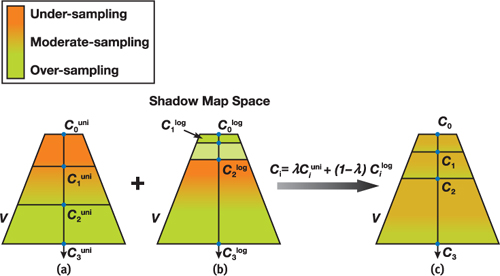$s(z)=\int_{0}^{s} d s=\frac{1}{\rho} \int_{n}^{z} \frac{1}{z} d z=\frac{1}{\rho} \ln \left(\frac{z}{n}\right)$

$s_{i}=s\left(C_{i}^{\log }\right)=\frac{1}{\ln (f / n)} \ln \left(\frac{C_{i}^{\log }}{n}\right) = {i \over m}\\ C_i^{\mathrm{log}} = n\left({f \over n}\right)^{i / m}$

$C_i^{\mathrm{uni}} = n + (f - n) {i \over m}$

## 第二步：计算光源的变换矩阵

1. 和场景无关的方法直接取完整包含分割块的部分作为 VP 矩阵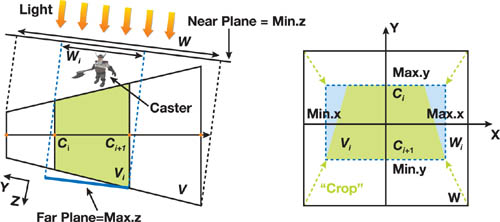2. 通过引入场景信息，可以只包含可能向视椎体中投影的所有物体即可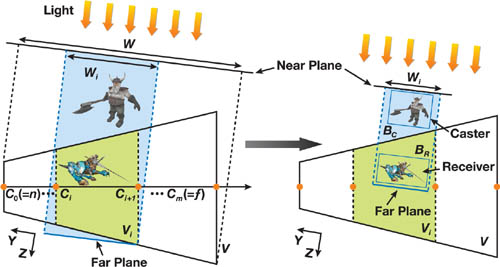## 第三、四步：生成 PSSM 并合成阴影

1. 多 pass 方法：这种方法是最通用的，但也由于没有利用硬件的加速从而效率较低
2. 利用 DX9 级别的硬件进行部分加速：这一方法可以去除生成阴影时需要的多 pass
3. 利用 DX10 级别的硬件进行完全加速：利用 geometry shader 的 cloning 和 instancing 进一步加速

# 基于硬件的实现方法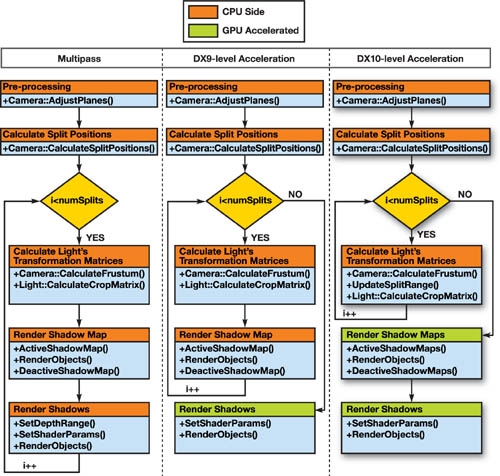## 准备阶段

### 使用 GS 的 Cloning 技术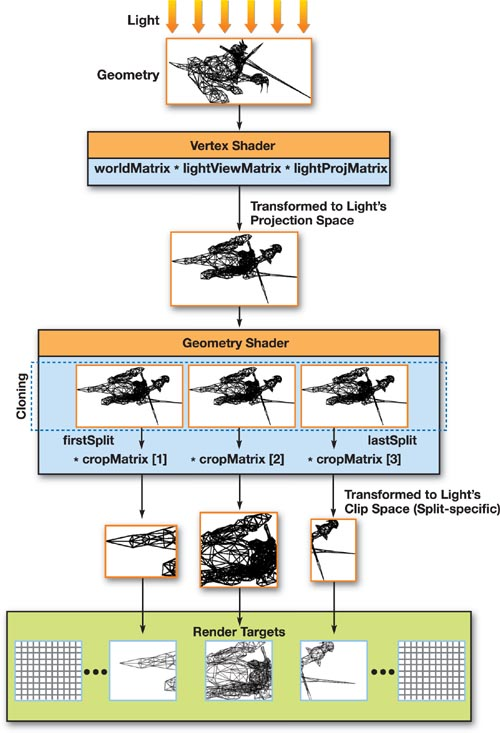### 使用 Instancing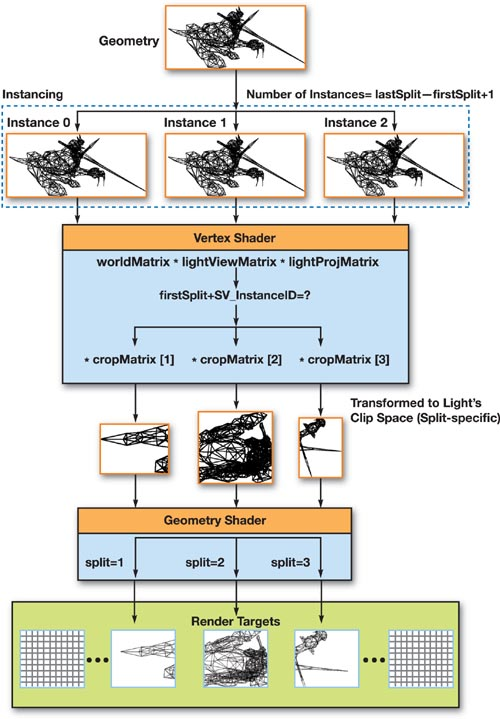# 更多的优化

• 滤波技术：使用 PCF，VSM 等方法对 SM 进行滤波以减少走样
• 打包材质：在材质采样器数量较少时，可以将不同的 SM 打包到一个材质内
• 结合不同的算法使用：一种有趣的分割算法的用途是在不同的分割内使用不同的 SM 方法，如 PSM，LiSPSM，TSM 等
• 可变的材质分辨率：在不同的分割内使用不同的分辨率以减少材质生成和储存的花费
• 在线性空间处理深度